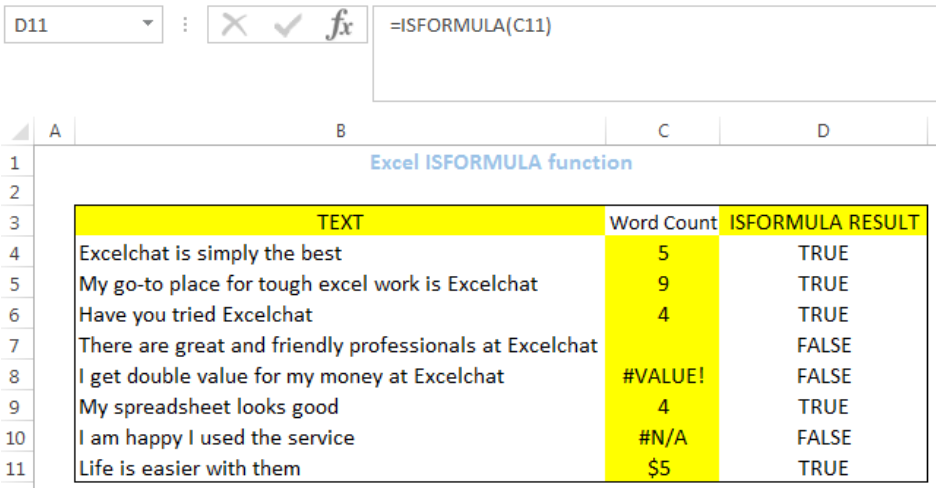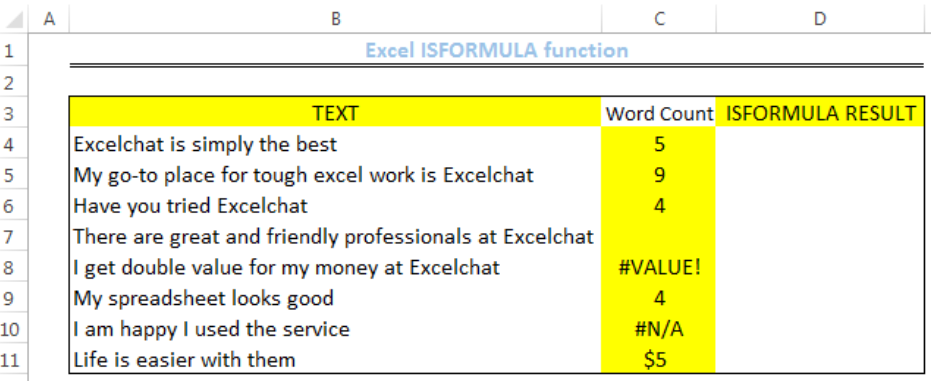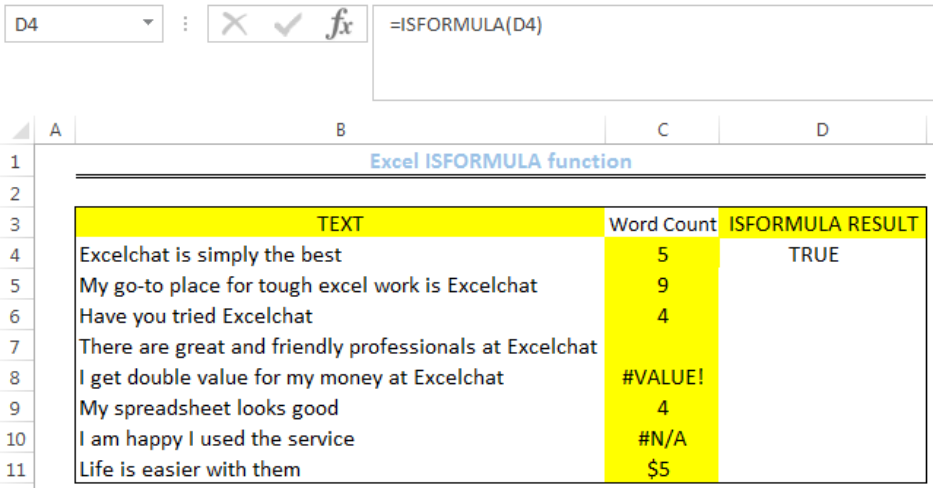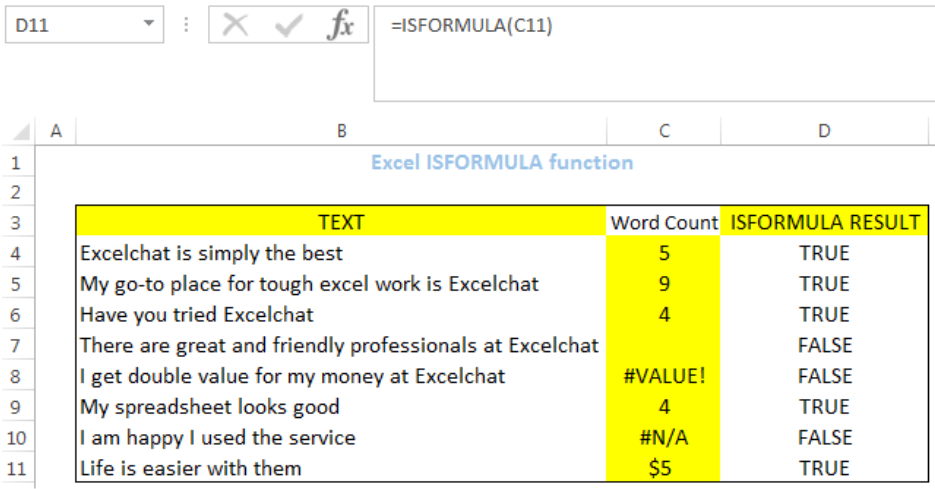Get instant live expert help with Excel or Google Sheets“My Excelchat expert helped me in less than 20 minutes, saving me what would have been 5 hours of work!”

#### Post your problem and you’ll get Expert help in seconds.

Your message must be at least 40 characters
Our professional Expert are available now. Your privacy is guaranteed.

# How to use the Excel ISFORMULA function

We can use the excel ISFORMULA function to check if a cell contains a formula or not. Even if the formula is valid or in an error state, the ISFORMULA function still returns TRUE if a formula is found and FALSE if otherwise. The steps below will walk through the process.Figure 1: How to use the Excel ISFORMULA function

## Syntax

`=ISFORMULA(reference)`

• Reference is the cell we want to check if it contains a formula

## Formula

`=ISFORMULA(D4)`

## Setting up the Data

• We have text values that are been counted. We will use the ISFORMULA FUNCTION to check if all the cells in Column C have the formula to count the TEXT in Column B
• We will set up the data by inputting the TEXT in Column B
• We will input the WORD COUNT into Column C
• Column D is where we want the ISFORMULA FUNCTION to return the resultFigure 2: Setting up the Data

## ISFORMULA Function

• We will click on Cell D4
• We will insert the formula below into the cell
`=ISFORMULA(D4)`
• We will press the enter keyFigure 3: Output for Cell D4 with the ISFORMULA Function

• We will click on Cell D4 again
• We will double-click on the fill handle (the small plus sign at the bottom right of Cell D4) and drag down to copy the formula into the other cellsFigure 4: Result for Column D with the ISFORMULA function

## Note

• The FORMULATEXT Function can be used to display the formula in COLUMN C

## Instant Connection to an Expert through our Excelchat Service

Most of the time, the problem you will need to solve will be more complex than a simple application of a formula or function. If you want to save hours of research and frustration, try our live Excelchat service! Our Excel Experts are available 24/7 to answer any Excel question you may have. We guarantee a connection within 30 seconds and a customized solution within 20 minutes.